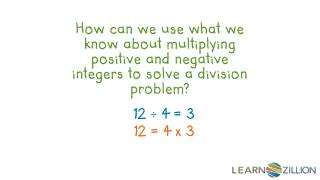# 12 ÷ 4 = 3 12 = 4 x 3 - PowerPoint PPT PresentationDownload Presentation12 ÷ 4 = 3 12 = 4 x 3

12 ÷ 4 = 3 12 = 4 x 3Download Presentation## 12 ÷ 4 = 3 12 = 4 x 3

- - - - - - - - - - - - - - - - - - - - - - - - - - - E N D - - - - - - - - - - - - - - - - - - - - - - - - - - -
##### Presentation Transcript

1. How can we use what we know about multiplying positive and negative integers to solve a division problem? 12 ÷ 4 = 3 12 = 4 x 3

2. In this lesson you will learn rules for dividing positive and negative integers by using the inverse relationship between multiplication and division.

3. x -4 = -20 -6 x (-3) = +18

4. Even though we are dividing two negative integers, the answer is not negative. -12 ÷ -4 = + 3

5. What do we mean by the inverse of multiplication and division? 3 x 5 = 15 15 ÷ 3 = 5 15 ÷ 5 = 3

6. Think about inverse relationship 18 ÷ 3 = ? 6 = 18 3 x ? 18 ÷ 6 = 3

7. Think about inverse relationship -2 x 5 = -10 -10 ÷ 5 = -2 -10 ÷ -2 = +5

8. Think about inverse relationship -16 ÷ 8 = ? -2 = -16 8 x ? -16 ÷ 8 = -2

9. Think about inverse relationship -3 x -4 = +12 +12 ÷ -3 = -4 +12 ÷ -4 = -3

10. Think about inverse relationship -20 ÷ (-4) = ? +5 = -20 ? -4 x -20 ÷ -4 = +5

11. In this lesson you have learned the rules for dividing positive and negative integers by using the inverse relationship between multiplication and division.

12. Find the quotient: -30 ÷ 6 = -28 ÷ (-4) = -5 7

13. Find the Quotient: -12 ÷ 4 = -40 ÷ (-10)= -27 ÷ (-9) = -10 ÷ 5 =